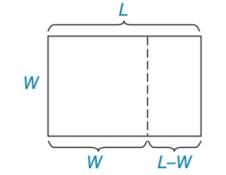Chapter 5.1, Problem 38E### Elementary Geometry for College St...

6th Edition
Daniel C. Alexander + 1 other
ISBN: 9781285195698

#### Solutions

Chapter
Section### Elementary Geometry for College St...

6th Edition
Daniel C. Alexander + 1 other
ISBN: 9781285195698
Textbook Problem
1 views

# To find the golden ratio (see the Discover activity on page 215), solve the equation 1 L = L − 1 L for L.(HINT: You will need the Quadratic Formula.)To determine

To find:

The solution of L.

Explanation

According to the quadratic formula, the solutions of ax2 + bx + c = 0 are given by

x=b+b24ac2a,bb24ac2a

Calculation:

Consider, 1L=L11

L(L1)=11L2L=1L2L1=0

Use the quadratic formula in L2L1=0

### Still sussing out bartleby?

Check out a sample textbook solution.

See a sample solution

#### The Solution to Your Study Problems

Bartleby provides explanations to thousands of textbook problems written by our experts, many with advanced degrees!

Get Started

#### Find more solutions based on key concepts